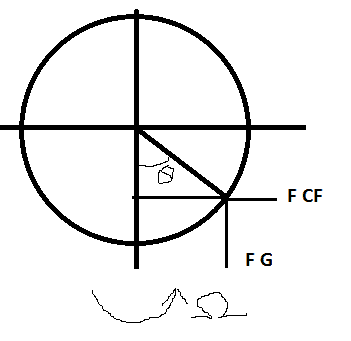# Help understanding centrefugal force

## Homework Statement

Basically i have to prove a lagrange equation using a non-inertial reference frame. A bead on a hoop where the hoop rotates as in the image below.F = Fg + Fcf

## Homework Equations

mR(d2θ/dt2) = mΩ2Rcosθsinθ -mgsinθ

what i'm supposed to get

## The Attempt at a Solution

Fg = -mgsinθ

Fcf = m(ΩxR)xΩ = mΩ2Rcosθsinθ

I know that

Ω = Ω(0 , sinθ, cosθ)

R = R( 0 ,

k i'm just lost and frustrated :(